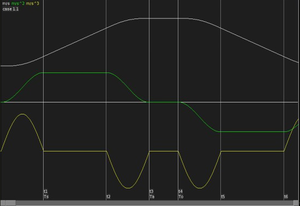Agar 1.7 Manual

M_Plotter(3)

# SYNOPSIS

```#include <agar/core.h>
#include <agar/gui.h>
#include <agar/math/m.h>
```

# DESCRIPTIONThe M_Plotter widget plots one or more numerical datasets. The widget is suitable for plotting data in real-time (fetching the data from different types of sources), but it can also plot existing datasets all at once. Extra annotations (labels) can be associated with the individual plots.

The data displayed by M_Plotter can be retrieved from different types of sources. Currently implemented sources include:
 M_PLOT_MANUALLY He data will be entered explicitely via calls to M_PlotReal() (see PLOTTING section). This is the default plot type set by M_PlotNew(). M_PLOT_FROM_VARIABLE_VFS Fetch the value of the given object variable/property. See AG_Object(3), AG_Variable(3). M_PLOT_FROM_REAL Fetch value by dereferencing a pointer to an M_Real. M_PLOT_FROM_INT Fetch value by dereferencing a pointer to an int. M_PLOT_FROM_COMPONENT Fetch value from entry i, j of a M_Matrix 3. M_PLOT_DERIVATIVE Compute as the derivative of another M_Plot.

# INHERITANCE HIERARCHY

AG_Object(3)-> AG_Widget(3)-> M_Plotter.

# INITIALIZATION

M_Plotter * M_PlotterNew (void *parent, Uint flags)

void M_PlotterSizeHint (M_Plotter *ptr, Uint w, Uint h)

void M_PlotterSetDefaultFont (M_Plotter *ptr, const char *face, int size)

void M_PlotterSetDefaultColor (M_Plotter *ptr, int colorIdx, Uint8 r, Uint8 g, Uint8 b)

void M_PlotterSetDefaultScale (M_Plotter *ptr, M_Real xScale, M_Real yScale)

The M_PlotterNew() function allocates, initializes, and attaches a new M_Plotter widget. Acceptable flags include:
 M_PLOTTER_HFILL Expand horizontally in parent container. M_PLOTTER_VFILL Expand vertically in parent container. M_PLOTTER_EXPAND Shorthand for M_PLOTTER_HFILL M_PLOTTER_VFILL|.

M_PlotterSizeHint() sets an initial preferred widget size in pixels.

M_PlotterSetDefaultFont() configures a default font face for use with plotter labels (see PLOT LABELS section).

M_PlotterSetDefaultColor() sets entry colorIdx in the palette of default plot colors. Newly created plots are assigned an initial plot color from this palette in a round-robin fashion. Valid indices are 0 up to M_PLOTTER_NDEFCOLORS-1.

M_PlotterSetDefaultScale() sets the default X and Y scaling factor that will be assigned to newly created plots.

# PLOTTING

M_Plot * M_PlotNew (M_Plotter *ptr, enum m_plot_type type)

M_Plot * M_PlotFromReal (M_Plotter *ptr, enum m_plot_type type, const char *label, M_Real *variable)

M_Plot * M_PlotFromInt (M_Plotter *ptr, enum m_plot_type type, const char *label, int *variable)

M_Plot * M_PlotFromDerivative (M_Plotter *ptr, enum m_plot_type type, M_Plot *plot)

M_Plot * M_PlotFromVariableVFS (M_Plotter *ptr, enum m_plot_type type, const char *label, void *vfsRoot, const char *varName)

void M_PlotClear (M_Plot *pl)

struct ag_window * M_PlotSettings (M_Plot *pl)

void M_PlotSetColor (M_Plot *pl, Uint8 r, Uint8 g, Uint8 b)

void M_PlotSetScale (M_Plot *pl, M_Real xScale, M_Real yScale)

void M_PlotSetXoffs (M_Plot *pl, int xOffs)

void M_PlotSetYoffs (M_Plot *pl, int yOffs)

void M_PlotReal (M_Plot *pl, M_Real v)

void M_PlotRealv (M_Plot *pl, Uint n, const M_Real *values)

void M_PlotVector (M_Plot *pl, const M_Vector *v)

void M_PlotVectorv (M_Plot *pl, Uint n, const M_Vector **values)

void M_PlotterUpdate (M_Plot *pl)

M_PlotNew() creates a new plot with no label and a source type of M_PLOT_MANUALLY (see DESCRIPTION). The type, argument can take on the values:
```enum m_plot_type {
M_PLOT_POINTS,		/* Individual points */
M_PLOT_LINEAR,		/* Linear interpolation */
M_PLOT_CUBIC_SPLINE,	/* Cubic spline interpolation */
M_PLOT_VECTORS		/* Vector arrows/cones */
};
```

The M_PlotFromReal() and M_PlotFromInt() variants create a plot which will be generated by dereferencing the value of an integer or real variable. The plot is assigned a specified label string by default.

M_PlotFromDerivative() creates a plot which will be computed as the derivative of plot. Nothing prevents plot from being a derivative plot itself.

M_PlotFromVariableVFS() creates a plot that will be generated by reading the value of a numerical AG_Object(3) variable. The object itself must be located under vfsRoot, and the varName string can take on the form "<object-name>:<variable-name>". See AG_Variable(3) for details.

M_PlotClear() erases the existing contents of a plot.

M_PlotSettings() constructs and displays a dialog which allows the user to change plot parameters (style, color, etc.), as well as to display the plot data in tabular format.

M_PlotSetColor() configures an alternate color for plot pl in RGB format.

M_PlotSetScale() configures an alternate horizontal and vertical scaling factor for plot pl.

The functions M_PlotSetXoffs() and M_PlotSetYoffs() set specific display X and Y offsets. Note that these offsets are bound to scrollbars and can be manipulated by the user.

The M_PlotReal() function enters an explicit value v in plot pl. M_PlotRealv() enters data from an array values, containing n entries.

M_PlotVector() enters data from an M_Vector(3). M_PlotVectorv() enters data from an array of n vectors.

The M_PlotterUpdate() routine updates all plots (except those using the M_PLOT_MANUALLY source type), effectively increasing the width of the plot display. This involves the dereferencing of associated variables (and possibly the evaluation of AG_Variable(3) functions for M_PLOT_FROM_VARIABLE_VFS). If scrolling mode is set (scrolling mode can be enabled by the user panning to the right edge of the display), the display is scrolled by one increment.

# PLOT LABELS

M_PlotLabel * M_PlotLabelNew (M_Plot *pl, enum m_plot_label_type type, Uint x, Uint y, const char *format, ...)

M_PlotLabel * M_PlotLabelReplace (M_Plot *pl, enum m_plot_label_type type, Uint x, Uint y, const char *format, ...)

void M_PlotLabelSetText (M_Plot *pl, enum m_plot_label_type type, Uint x, Uint y, const char *format, ...)

The M_PlotLabelNew() function creates a new label, associated with plot pl, and returns a pointer to the new label object. The type argument can take on the values:
 M_LABEL_X Associate label with an X value. A vertical alpha-blended line will be rendered along with the label. M_LABEL_Y Associate label with an Y value. M_LABEL_FREE Label can be freely moved by the user.

The M_PlotLabelReplace() variant searches for an existing label with the same text string. If such a label is found, it is replaced by the new label.

M_PlotLabelSetText() changes the text string associated with the label. format is a standard format string.

# EVENTS

The M_Plotter widget does not generate any event.

# STRUCTURE DATA

For the M_Plotter object:
 int xOffs, yOffs Display offset in pixels (bound to scrollbars). M_Real xScale, yScale Horizontal and vertical scaling factors (also user-controlled). AG_Scrollbar *hbar Horizontal scrollbar object. AG_Scrollbar *vbar Vertical scrollbar object.www.libAgar.org is © 2022 Julien Nadeau Carriere <vedge@csoft.net>.